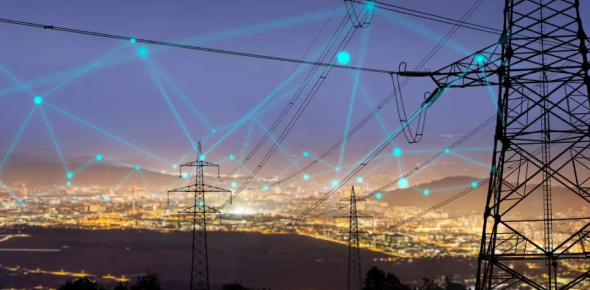# Electricity Review

20 Questions | Total Attempts: 260Settings.

• 1.
Circuit with more than one path
• A.

Parallel

• B.

Series

• 2.
A material that current does not easily flow through
• A.

Conductor

• B.

Insulator

• C.

Electric discharge

• D.

Voltage

• 3.
Rapid movement of excess electrons from one place to another
• A.

Static electricity

• B.

Insulator

• C.

Electric discharge

• D.

Series

• 4.
Rate at which electric energy is converted to another form
• A.

Electric field

• B.

Static charge

• C.

Circuit

• D.

Power

• 5.
Closed path through which a current can flow
• A.

Circuit

• B.

Voltage

• C.

Resistance

• D.

Conductor

• 6.
Buildup of electrical charges in one place.
• A.

Current electricity

• B.

Ohm's Law

• C.

Static charge

• D.

Electrical power

• 7.
Exists around every electric charge
• A.

Insulator

• B.

Electric field

• C.

Voltage

• D.

Electric discharge

• 8.
• A.

Static charge

• B.

Current

• C.

Electric field

• D.

Voltage

• 9.
Measure of how difficult it is for electrons to flow
• A.

Series

• B.

Resistance

• C.

Conductor

• D.

Static charge

• 10.
A material that current flows through easily
• A.

Conductor

• B.

Insulator

• C.

Electric discharge

• D.

Parallel

• 11.
An atom with a positive or negative charge
• A.

Electric field

• B.

Resistance

• C.

Parallel circuit

• D.

Ion

• 12.
A circuit with more than one path along which current can flow
• A.

Series

• B.

Parallel

• C.

Electric discharge

• D.

Resistance

• 13.
Place a checkmark beside all the following that are good conductors
• A.

Dry skin

• B.

Water

• C.

Rubber

• D.

Iron

• E.

Copper

• F.

Styrofoam

• 14.
Place a checkmark beside all of the insulators
• A.

Iron

• B.

Rubber

• C.

Dry skin

• D.

Copper

• E.

Styrofoam

• F.

Water

• 15.
Which of these things does a circuit need to work properly?
• A.

Bulb

• B.

A power supply

• C.

Crocodile clips

• D.

Plastic

• 16.
If a circuit is not complete
• A.

It will not work

• B.

It might work

• C.

It will work

• D.

The bulbs glow

• 17.
What do cells (batteries) do in a circuit
• A.

Nothing

• B.

Light up

• C.

Provide electricity

• D.

Use up electricity

• 18.
Will the following series circuit work?
• A.

Yes, it is a complete circuit.

• B.

No, it is not a complete circuit.

• 19.
Will the following LED light light up?
• A.

Yes, it is a complete circuit

• B.

No, the switches are open

• C.

No, the switches are closed

• 20.
Why do circuits need to be complete?
• A.

To make them round in shape

• B.

So the wires are all the same size

• C.

To make the bulbs go out

• D.

So that the electricity can travel all the way around

Related Topics